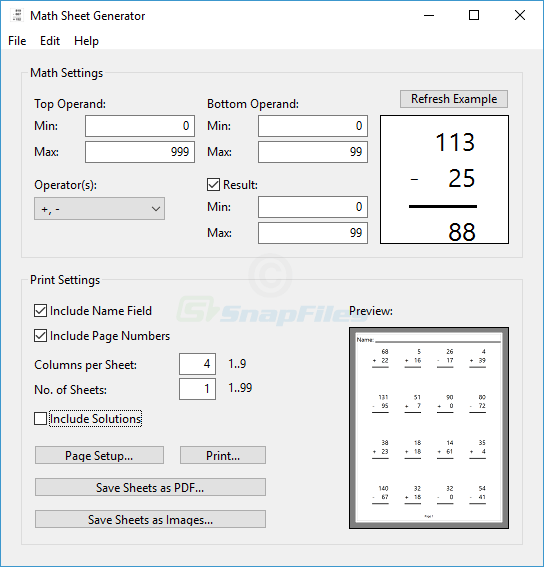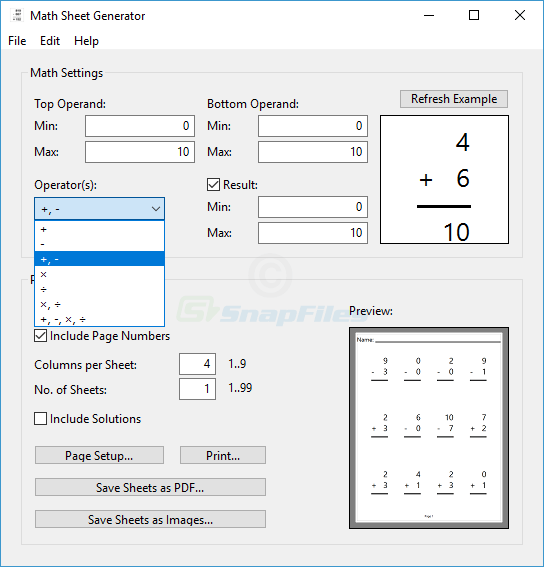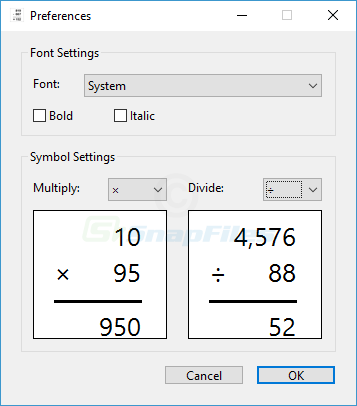# MathSheetGenerator screenshots

## create sheets of simple math exercises

MathSheetGenerator is a simple utility for educators and parents to automatically create sheets of simple math exercises for elementary school children. The program can generate math problems with two operands using any of the standard operators (add, subtract, multiply, divide) and you can set... [Read more...]Back to MathSheetGenerator